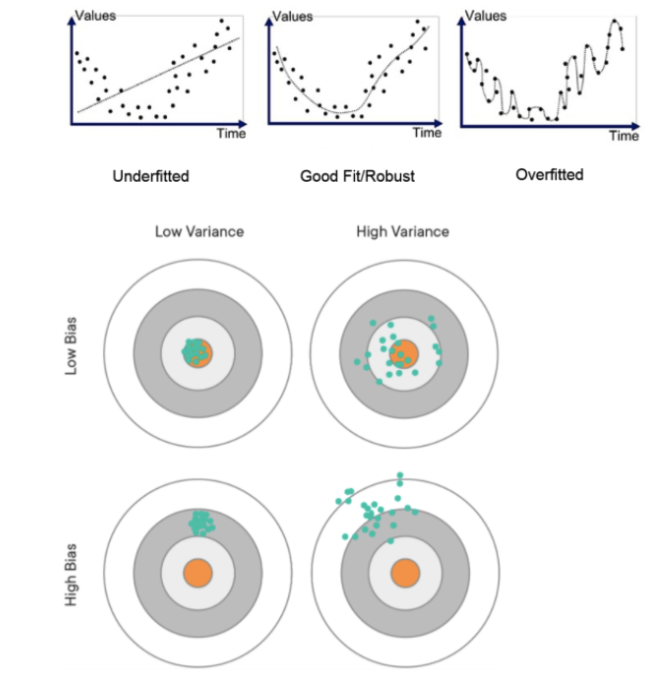# Multiple Linear Regression

kobeisfree94·2022년 4월 19일
0

Main Purpose: Building a model that accurately predicts the test data (as opposed to the train data)

Train/Test Split

• Train Data - used to train the model
• Test Data - used to check performance of the model
*** The two must be split in order to prevent data leakage

Simple Linear Regression vs. Multiple Linear Regression

• Simple Linear Regression
- 1 Feature/Dimension/Independent Variable
• Multiple Linear Regression
- 2+ Feature/Dimension/Independent Variable

Implementation:

• Uses the same fit_transform/transform in the sklearn.linear_model LinearRegression()
• Uses the same model.intercept, model.coef
• Difference: Uses 2 or more features

Evaluation Metrics:

• Mean Squared Error(MSE)
• Mean Absolute Error (MAE)
• Root Mean Sqaured Error (RMSE)
• R-squared (Coefficient of Determination)

Overfitting vs. Underfitting

• Key words:
1. Generalization - a model that returns high performance in both the train and test data.
2. Overfitting - a model that relies too heavily on the train data and thereby creates a difference/error in generalization
3. Underfitting - a model that hasn't been able to overfit or generalize. High chance of bias.